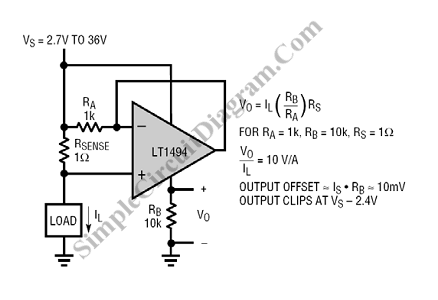# Self Powered Current Sensing

This is a current sensing circuit that senses the current at the high side (between load and positive supply). This circuit     take advantage from Rail-to-Rail input of the LT1494 and the microampere supply current. Here is the circuit :At the op-amp, the output is connected directly to the inverting input that will keep the inverting input is equal to the the noninverting input. So, the voltage across RA will be kept in the same level with the voltage across Rsense. The current flows  from power source to the output pin of the op-amp through RA and return to ground by RB because of this voltage. The output at RB is valid because almost all of the current at RB comes from RA and the internal circuit draws only very small amount of current through Vcc pin. [Circuit schematic diagram source: Linear Technology Application Notes]